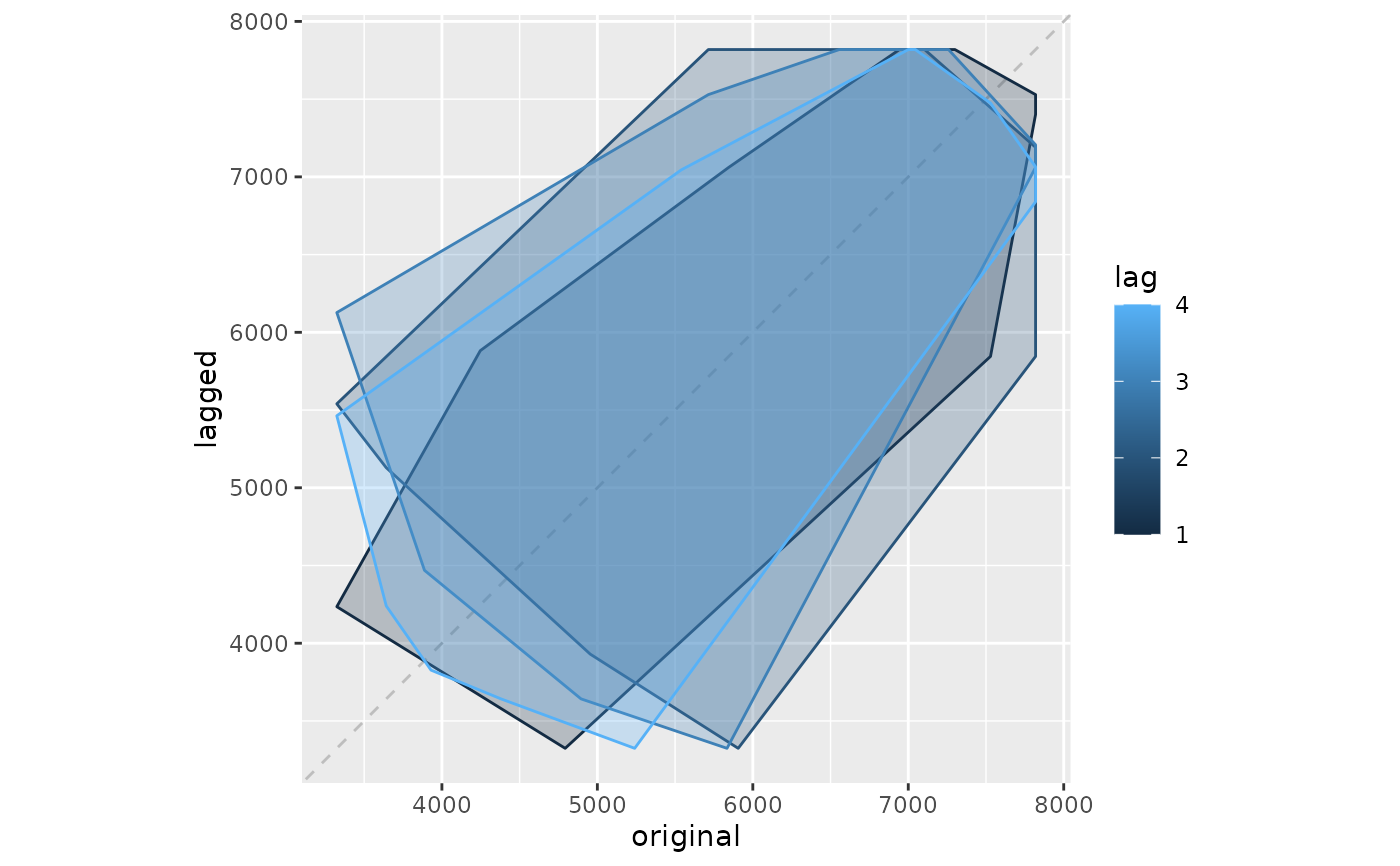Plots a lag plot using ggplot.

gglagplot(
x,
lags = ifelse(frequency(x) > 9, 16, 9),
set.lags = 1:lags,
diag = TRUE,
diag.col = "gray",
do.lines = TRUE,
colour = TRUE,
continuous = frequency(x) > 12,
labels = FALSE,
seasonal = TRUE,
...
)

gglagchull(
x,
lags = ifelse(frequency(x) > 1, min(12, frequency(x)), 4),
set.lags = 1:lags,
diag = TRUE,
diag.col = "gray",
...
)

## Arguments

x a time series object (type ts). number of lag plots desired, see arg set.lags. vector of positive integers specifying which lags to use. logical indicating if the x=y diagonal should be drawn. color to be used for the diagonal if(diag). if TRUE, lines will be drawn, otherwise points will be drawn. logical indicating if lines should be coloured. Should the colour scheme for years be continuous or discrete? logical indicating if labels should be used. Should the line colour be based on seasonal characteristics (TRUE), or sequential (FALSE). Not used (for consistency with lag.plot)

None.

## Details

“gglagplot” will plot time series against lagged versions of themselves. Helps visualising 'auto-dependence' even when auto-correlations vanish.

“gglagchull” will layer convex hulls of the lags, layered on a single plot. This helps visualise the change in 'auto-dependence' as lags increase.

lag.plot

## Author

Mitchell O'Hara-Wild

## Examples


gglagplot(woolyrnq)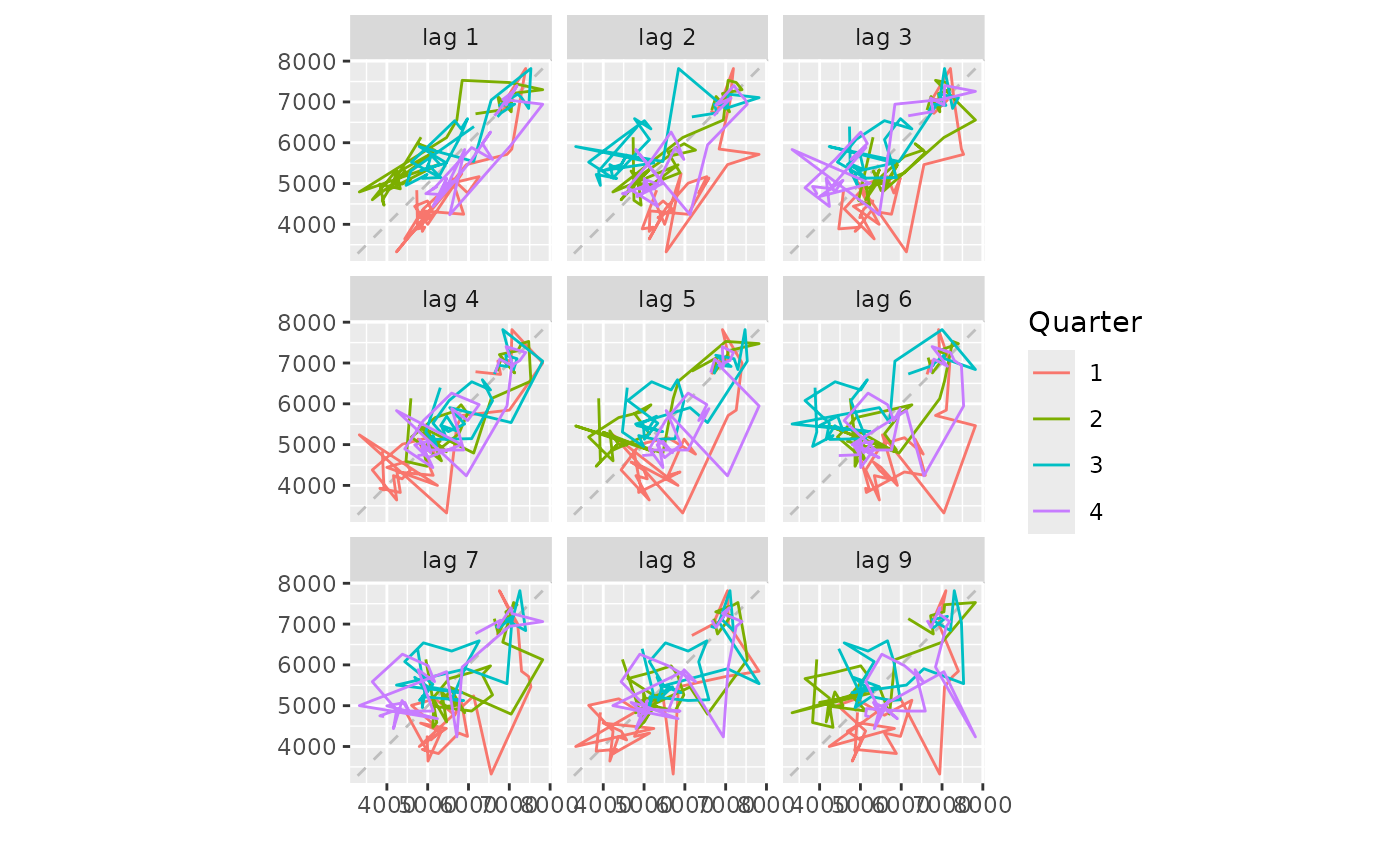gglagplot(woolyrnq,seasonal=FALSE)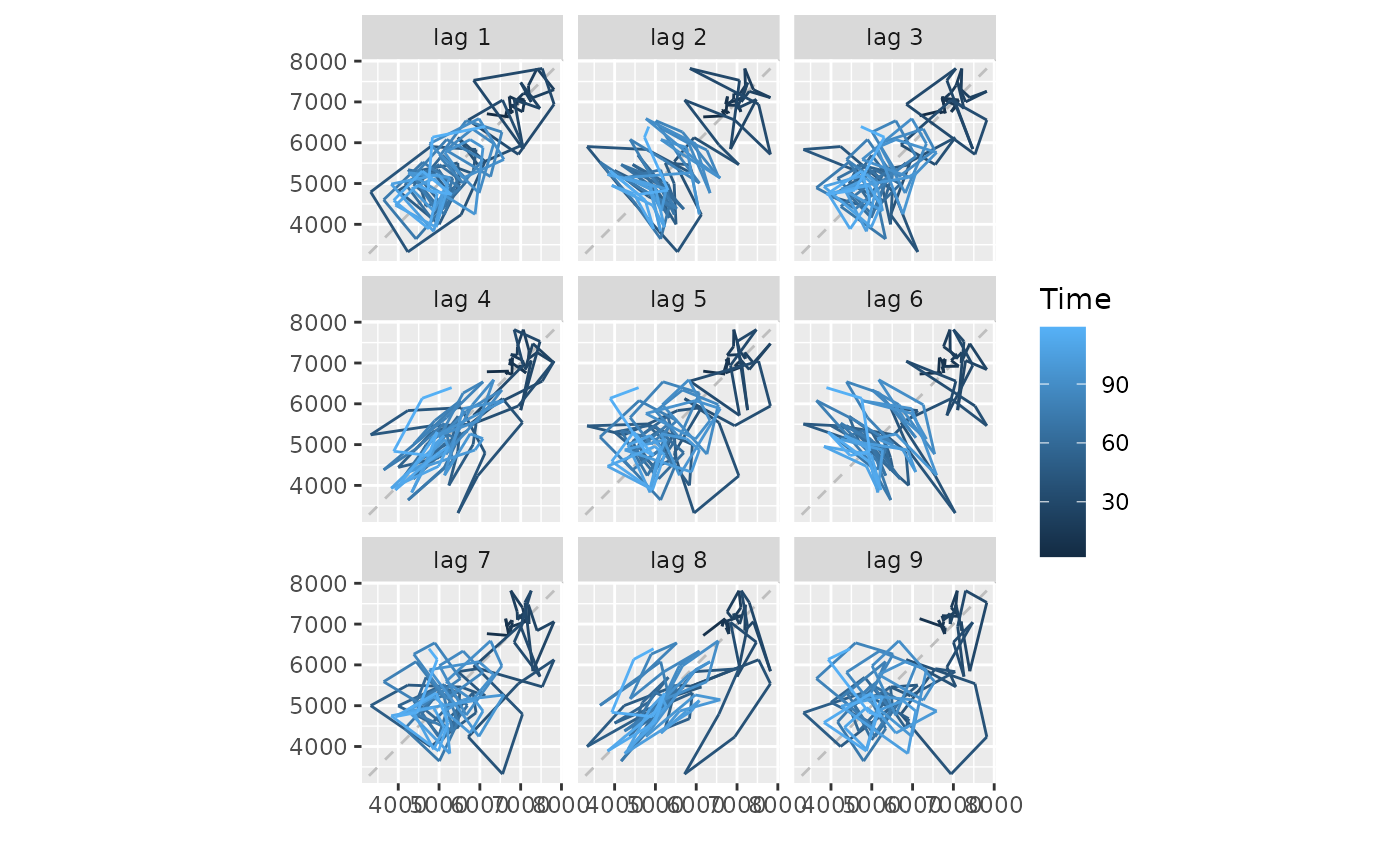lungDeaths <- cbind(mdeaths, fdeaths)
gglagplot(lungDeaths, lags=2)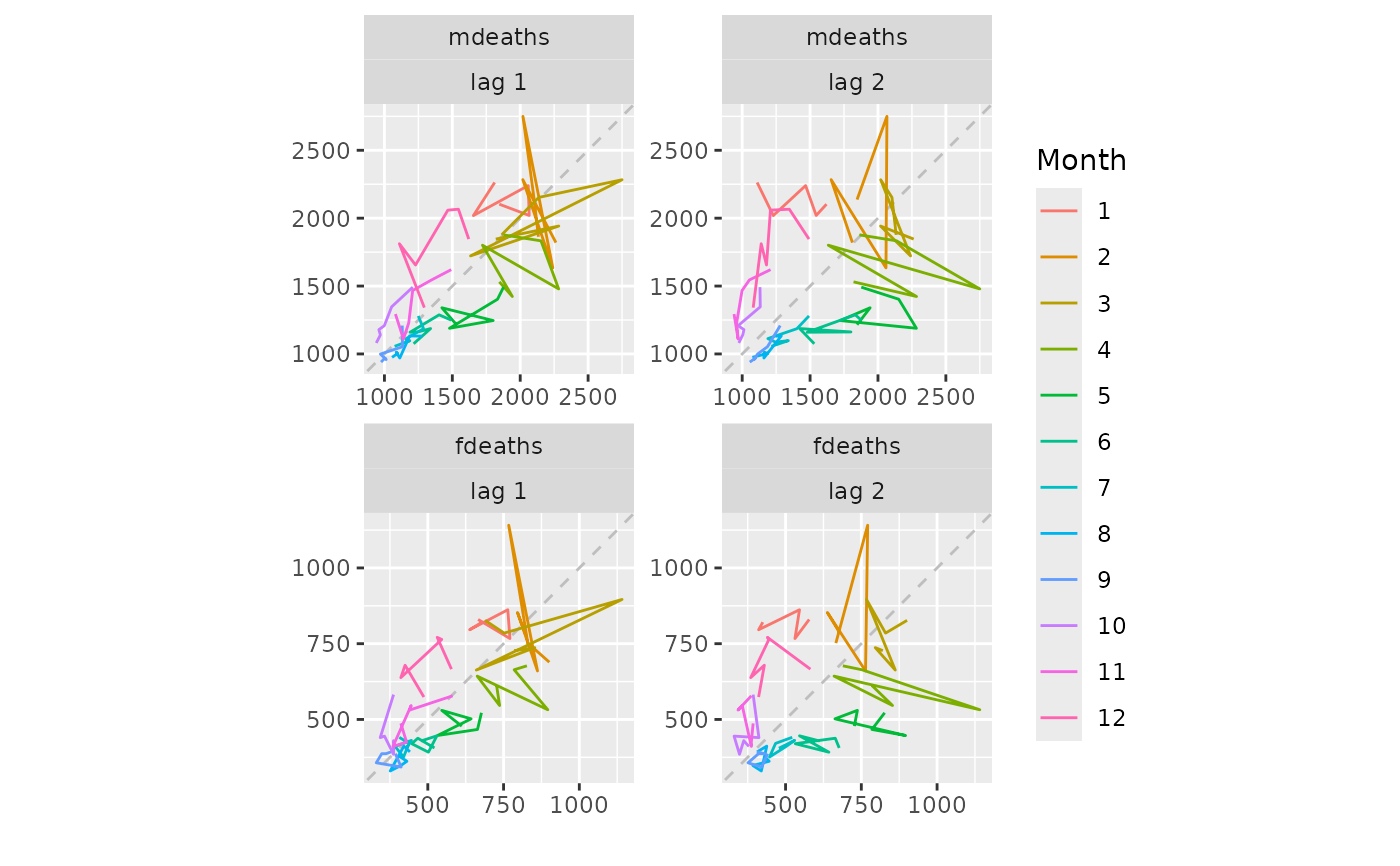gglagchull(lungDeaths, lags=6)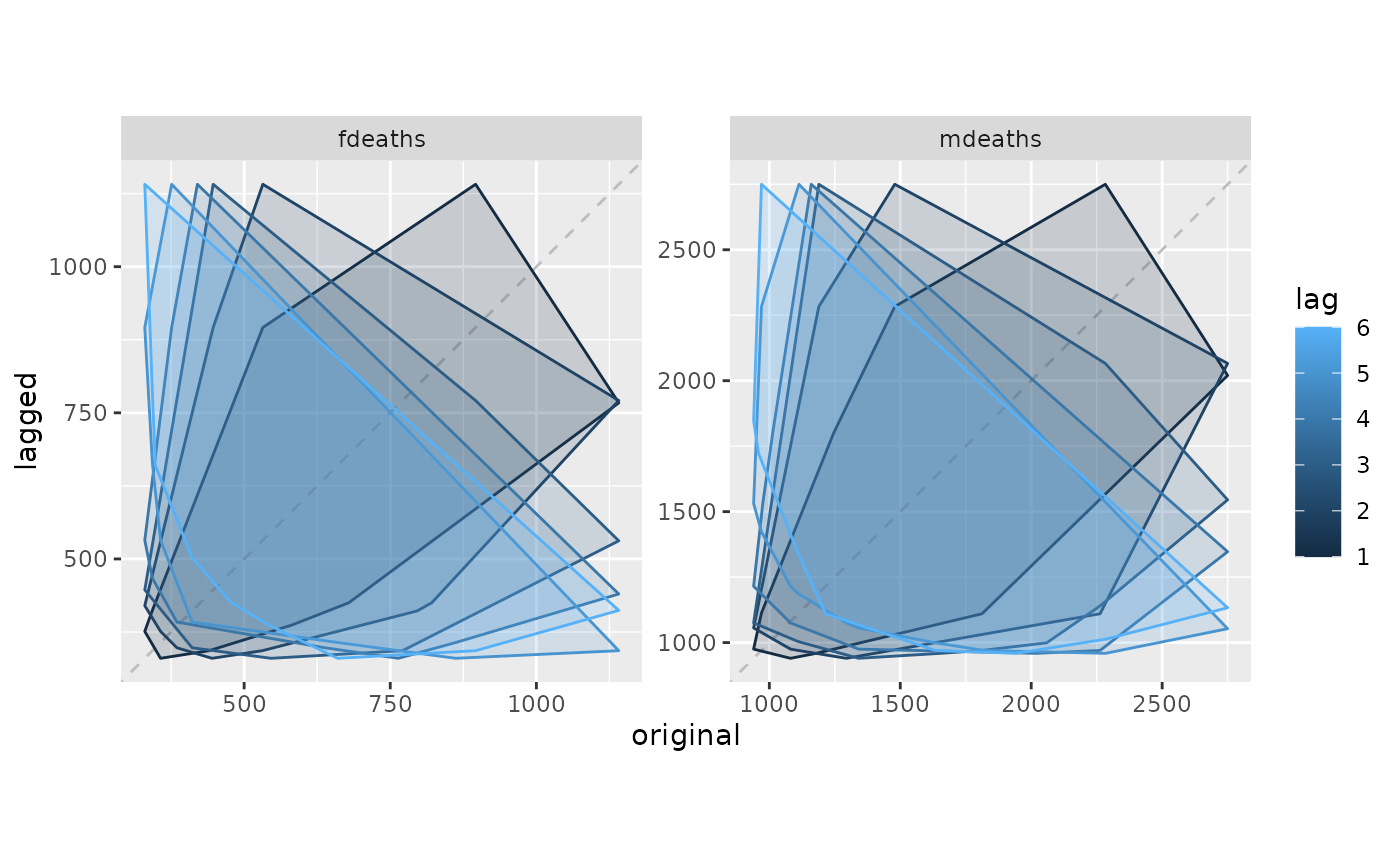gglagchull(woolyrnq)